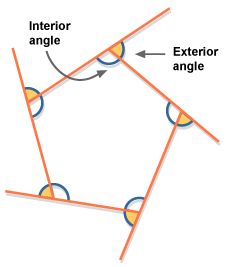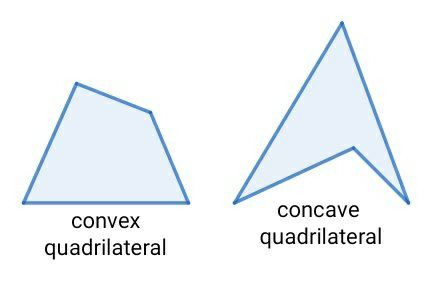Courses

# Understanding shapes 2 (Quadrilaterals)class8 Class 8 Notes | EduRev

## Class 8 : Understanding shapes 2 (Quadrilaterals)class8 Class 8 Notes | EduRev

The document Understanding shapes 2 (Quadrilaterals)class8 Class 8 Notes | EduRev is a part of Class 8 category.
All you need of Class 8 at this link: Class 8

Definition-let a, b ,c and d are the four points in a plane such  that-

1. No three of them are collinear

2. The line segment ab BC CD and DA do not intersect except at their and points.

Sides-in a quadrilateral ABCD the four line segment AB, BC , CD and DA  ,are called its side.

Adjecent Sides-Two sides of a quadrilateral are called its adjacent sides , if they have a common end points.

Opposite sides-Two sides of a quadrilateral are called its opposite sides if they do not have a common end point.

Diagonals- In the quadrilateral ABCD the line segment AC and BD are called its diagonal.

Angles- In the quadrilateral ABCD the angles, angle DAB , angle ABC angle BCD and angle CDA are called its angle. These angles are denoted by angle a angle b angle c and angles respectively.

Adjacent angle-two angles of a quadrilateral are called Adjacent angle, is they have a common side as an arm.

Interior and exterior of a quadrilateral.Figure 1-shows interior and exterior angle of a quadrilateral.

Definition-a quadrilateral is called convex quadrilateral if the line containing any side of the quadrilateral has the remaining vertices on the same side of it.Figure 2

Convex and concave quadrilateral shows in figure 2

I hope this helps you!!!

Thank u!!!!!

Offer running on EduRev: Apply code STAYHOME200 to get INR 200 off on our premium plan EduRev Infinity!

,

,

,

,

,

,

,

,

,

,

,

,

,

,

,

,

,

,

,

,

,

;